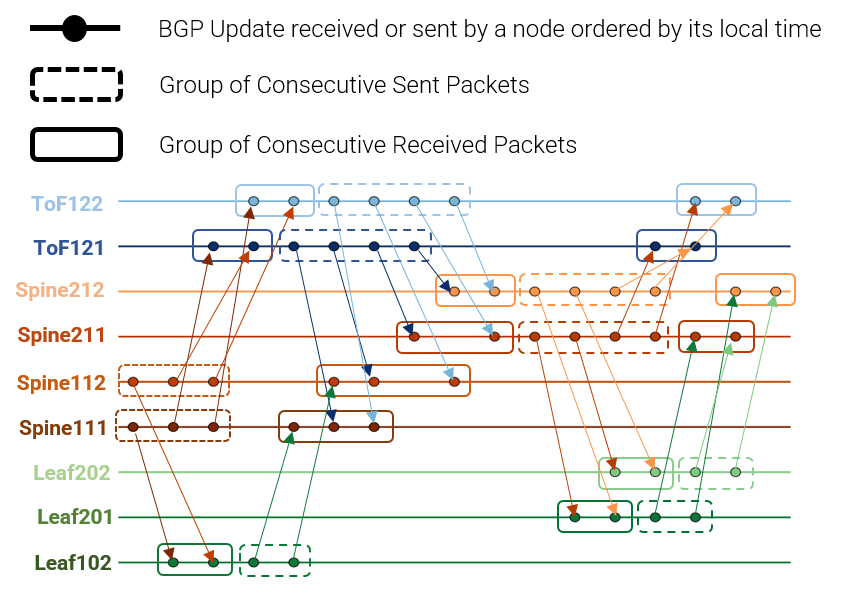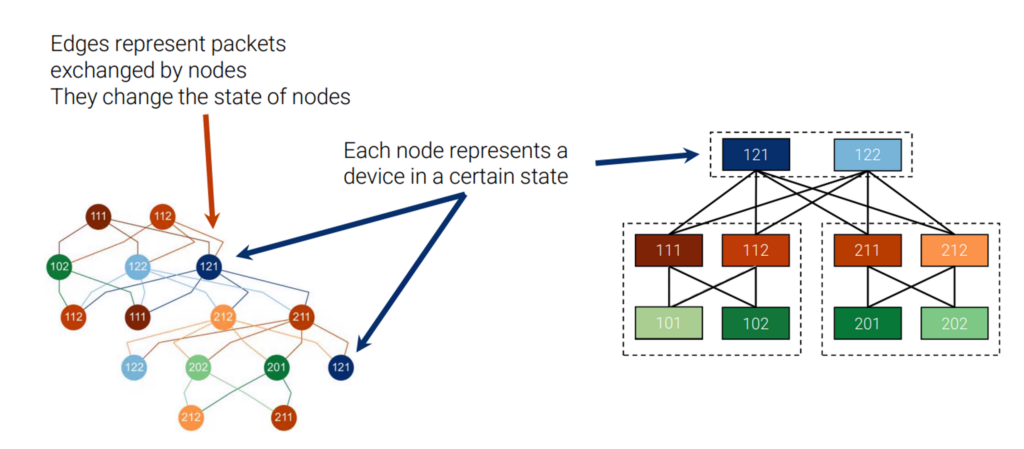# Sibyl: A framework for evaluating the implementation of routing protocols in fat-trees

By on 17 Feb 2023

Category: Tech matters

Tags: , , ,Data centres are a critical part of the Internet infrastructure as they host a wide range of services, guaranteeing efficient deployment and low latencies. These features come with the cost of more complexity in the network, which typically translates to higher levels of node density and in a wide variety of heterogeneous technologies, protocols and implementations.

In this context, we need efficient testing procedures and metrics to ensure that all the elements behave as expected. Unfortunately, there is a lack of standard testing procedures for routing protocols, which represents one of the fundamental building blocks of the networks.

In this post, my fellow researchers from Roma Tre University and Universidad de la República and I illustrate Sibyl, a framework developed trying to fill this critical gap.

## We need more tests

Someone could argue: ‘Why more tests? The Internet works!’. Well, the answer is easy — we need them. In fact, several Over-The-Top (OTT) media services state that a considerable amount of failures in their data centres are caused by configuration errors and software bugs (for example, CrystalNet). The main reason is that testing these complex networks is a difficult task, which requires carefulness and some preliminary reasoning.

## The need for emulation

Performing tests on production networks is not feasible since they could impact hosted services, violating agreements (for example, service level agreements) and affecting end users. So, the first idea that could come to mind is to build a simulation of the production network, where one can test deployment and protocol behaviours at scale. However, this is not an option if our purpose is to evaluate real protocol implementations. In fact, simulation assumes an ideal bug-free environment where real software bugs and configuration errors cannot be detected.

For these reasons, we think that emulation is needed to faithfully test software behaviours.

The major drawback of this choice is that an emulated environment cannot consider time-based metrics, since they heavily depend on the used hardware and can result in misleading conclusions.

So, as the first step, we designed a methodology that adopts a wall-clock independent method to establish metrics, allowing normalization of the results independently from the execution environment. Then we developed Sibyl, a framework that, implementing the methodology, leverages emulation to test routing protocol implementations on fat-trees in an automatic, scalable and reliable way. We focus on fat-trees since they are the most common data centre topology used today, but the idea can be generalized for other ones.

## The Sibyl Framework: A practical example

Let’s see how Sibyl works with a practical example (refer to the original paper for a formal explanation of the methodology and how the framework works internally).

Before starting we need to clarify some points:

1. For describing a fat-tree, we adopt the terminology of the Routing In Fat-Trees (RIFT) protocol, currently under standardization at the IETF. You can find the most important definitions in Figure 1.
2. We use Kathará as the underlying network emulator, which we chose because it can leverage a Kubernetes cluster for performing tests on hyper-scale topologies.

Now, let’s consider a simple fat-tree like the one in Figure 1.

Suppose that we want to test and analyse how the FRR BGP implementation (Sibyl currently also supports FRR IS-IS, FRR Openfabric, and RIFT-Python) behaves in case of a Leaf failure. We only need to tell Sibyl to perform an experiment using FRR BGP on the desired topology.

At a high level, Sibyl performs the following steps:

1. It generates the desired topology configuration for Kathará.
2. It uses Kathará to deploy the topology and deploys an agent service on each node to interact with them.
3. It starts dumping traffic on all the links of the network.
4. It starts the routing protocol (FRR BGP) and waits for its convergence, checking that the nodes’ forwarding tables reach the expected state. If the check fails after a maximum number of attempts, the test is aborted.
5. It performs the event (the failure of Leaf101).
6. Again, it waits for the convergence of the protocol, checking that the nodes’ forwarding tables reach the expected state after the failure. As above, if the check fails after a maximum number of attempts, the test is aborted.
7. It stops the dumps and collects the results.
8. It uses Kathará to undeploy the topology.

After an experiment (or a set of experiments), we can use Sibyl’s analysis tools to investigate the protocol implementation behaviour.

During the analysis, we consider the following aspects:

Messaging Load: The aim is to evaluate how the protocol overhead grows with respect to the topology parameters (for example, the number of ports of the switches, and the value of the redundancy factor). To do this, we count the packets originated by a test and compute their aggregate size.

Now, let’s consider Figure 2 (automatically produced by Sibyl), which shows the number of packets exchanged after Leaf101 failure in our experiment. We can see two packets on each link, which result in a total value of 28 for the Messaging Load.

Locality: To evaluate the ability of an implementation to limit the ‘blast radius’ of a failure or a recovery event, Sibyl leverages the Locality metric, which is computed as follows: ​

1. We count the number of packets that traverse each link of the network during an event.
2. We assign a topological distance to each link, computed as the distance from the event.
3. We compute the total number of packets received at a certain topological distance.
4. The result is a vector where each element is the number of packets captured at a specified distance. We summarize it into a scalar value giving a larger weight to packets that are far from the event.​

Figure 3 shows that the value of the Locality in our experiment is equal to 60 and how it is computed.

Rounds: The way Locality is considered allows us to perceive the ‘blast radius’ of an event but it doesn’t give any information about the number of ’rounds’ when this happens. Packets could be received all at the same time or at different timing. However, considering this aspect being oblivious as much as possible with respect to the actual timing is a challenging task. To do that, we introduce a novel metric called ‘Round’.Figure 4 — The node-state timeline of the FRR BGP implementation in case of the failure of Leaf101.

To analyse the round aspect we introduce two novel representations — the node-state timeline and the node-state graph.

The node-state timeline shows the interaction between nodes. It is computed by ordering, for each node of the network, the packets dumped during an experiment on a timeline and linking each sent packet of a transmitter node with its corresponding received packet on the receiver node. Figure 4 shows the node-state timeline related to the Leaf-node failure of our example. We use this representation to ensure the correctness of the interactions between nodes and to quantify the total amount of interactions needed for the protocol to converge.Figure 5 — The node-state graph of the FRR BGP implementation in case of the failure of Leaf101 (only 101 on the fat-tree).

From the node-state timeline we construct the node-state graph (the formal process described in the original paper), which allows us to analyse the number of state changes the network traverses before the convergence. Figure 5 shows the node-state graph of our example. Each node of the graph represents a node of the network in a certain state. While each edge represents a set of packets from the upper node that contributes to changing the state of a lower node. The graph gives us an overall view of the interactions between nodes, where the root nodes are the first to detect the failure of Leaf101, and the nodes at the lower levels that are the last to be notified.

Using this representation, we can:

1. Compute the overall number of state changes in the network.
2. Compute for each vertex the number of rounds, as the length of the longest path from a source to that vertex, needed for reaching the convergence state. We call this measure the state-round value of a vertex.​
3. Compute the maximum value among the state-round values of the vertices. We call this state-round value of the graph, which tells us the number of rounds that an implementation needs to converge after an event. In our example, it is easy to compute that the maximum length of a path in the graph is 4, so the rounds needed by FRR BGP to converge after a leaf failure is 4.

As you noticed, all these metrics do not consider time and so can be safely used in an emulated environment.

This is only an example of usage, but what use cases can be implemented on top of a framework like Sibyl?

## The Sibyl Framework: Use cases

Evaluating protocol implementations: A first possible use case is the evaluation of a protocol implementation to analyse how it behaves in various operational scenarios and if it scales up with the topology. In fact, thanks to the possibility to run the experiments in a Kubernetes cluster, Sibyl allows us to also test hyper-scale scenarios (even in a public cloud). In the original paper, we show a complete evaluation of the FRR BGP implementation on fat-trees up to 1,280 nodes and more than 33k interfaces.

Comparing protocol implementations: Sibyl enables us to test different protocol implementations and compare the results in terms of messaging load, locality and rounds. This opens the possibility to analyse the implementations’ behaviour and clearly state in which scenarios they perform better. Moreover, since our metrics do not consider temporal-tied aspects, allowing normalization of the results enables the comparison of implementations that are quite different from each other and of prototypes. We show an example of this comparison in the paper.

Supporting the implementation and debugging of protocols: Sibyl allows developers to build a testing pipeline in order to test and evaluate code changes during development.

In particular, the node-state graph and the timeline could be very useful in checking the correctness of the interactions between nodes and ensuring that the overall behaviour is the one expected.

We personally experimented with this, exploiting Sibyl for implementing a feature (that is, the negative disaggregation) in the RIFT-Python implementation and analysing the Juniper implementation of RIFT, as described in the original paper.

Other use cases: Sibyl provides a set of APIs and base templates to extend the implemented scenarios. This allows users to define custom scenarios that can be used to implement other use cases like interoperability tests or checking network protocol configurations before deploying changes in the production network.

## Conclusions

In this post, we saw why we need more accurate and standard testing procedures and how we try to address this issue. The Sibyl methodology is devised for fat-trees, but it can be easily extended to support other topologies or experiments. With this work, we aim to give the community standard testing methodologies and open-source tools to test, evaluate and compare routing protocol implementations.

You can find more formal descriptions of the methodology and framework in the original paper.

All the code base is publicly available on GitLab; feel free to play with it and contact us if needed.

This post was co-authored by Mariano Scazzariello. I would also like to thank all the paper co-authors: Mariano Scazzariello, Leonardo Alberro, Lorenzo Ariemma, Alberto Castro, Eduardo Grampin and Giuseppe Di Battista.

Tommaso Caiazzi is a PhD student of Computer Networks in the ‘Computer Networks and Security’ research group at the Roma Tre University. His research interests are data centre networks, mainly focusing on routing protocols and high-speed networking.